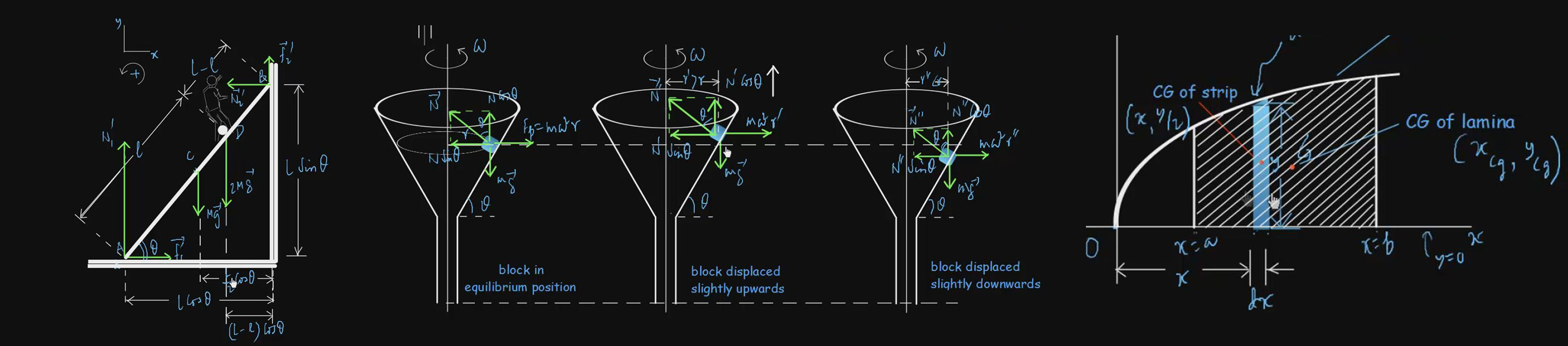# Motion In One and Two Dimensions

Mechanics can be divided into two parts: dynamics and statics. Dynamics is the study of motion of a body under one or more forces. Statics is the study of the condition of rest of a body under a number of forces. Dynamics is further divided into kinematics and kinetics. Kinematics is that part of dynamics which deals with motion without reference to the forces that cause it or the properties of the body in motion. Kinetics is that part which relates the motion of a body to its mass and the casual force(s). The subtopics covered in the present topic such as Projectile Motion, Translational Motion, Graphical Analysis of Rectilinear Motion, Rectilinear Motion with Constant Acceleration, Rectilinear Motion under Gravity etc. fall within the scope of kinematics.

#### Basic level videos

Analysis Of Rectilinear Motion By Calculus 30:46 Basic
200 3
Graphical Analysis Of Rectilinear Motion 57:17 Basic
300 5
Kinematic Equation By Integration 28:46 Basic
200 3
Kinematic Equations By Graphical Method 23:29 Basic
200 3
Motion Of A Boat In A River 58:10 Basic
300 5
Problems On Kinematic Equations 1:02:21 Basic
350 7.5
Problems On Rectilinear Motion Under Gravity 27:35 Basic
200 7.5
Problems On Relative Velocity And Relative Acceleration 1:53:39 Basic
400 8
Projectile Motion 1:02:33 Basic
350 7.5
Rectilinear Motion Under Gravity 50:08 Basic
300 5
Rectilinear Motion With Constant Acceleration 31:45 Basic
200 3
Relative Velocity And Relative Acceleration 38:57 Basic
200 3
Rest And Motion 56:19 Basic
300 5
Some Terms Associated With Translational Motion 1:18:15 Basic
350 7.5

#### Advanced level Videos Note: (CE) Stands for Problems from Competitive Examination Papers

Advanced-Level Problems On Motion In One And Two Dimensions I 1:05:48
300
5
Advanced-Level Problems On Motion In One And Two Dimensions II 1:05:03
350
7.5
Advanced-Level Problems On Motion In One And Two Dimensions III 1:11:12
350
7.5
Advanced-Level Problems On Motion In One And Two Dimensions IV 1:01:05
350
7.5
Advanced-Level Problems On Motion In One And Two Dimensions VI 1:08:18
350
7.5
Problems On Motion In One And Two Dimensions (CE) 1:21:28
350
7.5
Problems On Motion In One And Two Dimensions II (CE) 1:14:11
350
7.5